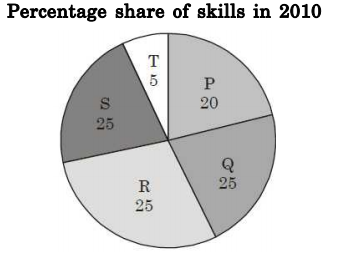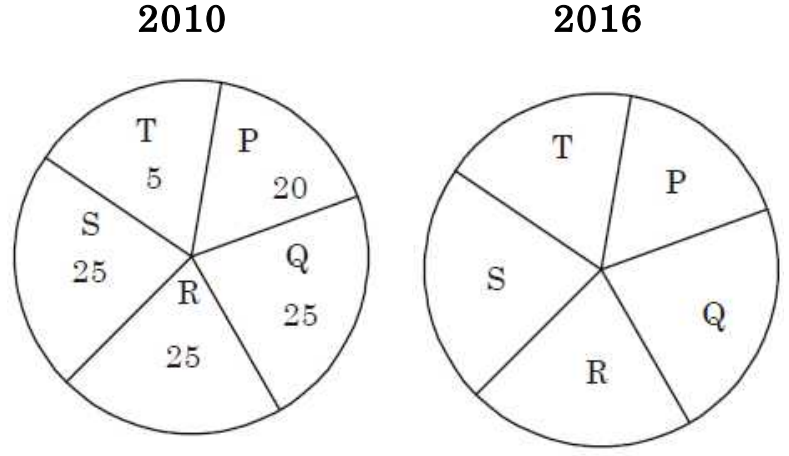## Numerical Ability

#### Numerical Ability

1. A firm hires employees at five different skill levels P, Q, R, S, T. The shares of employment at these skill levels of total employment in 2010 is given in the pie chart as shown. There were a total of 600 employees in 2010 and the total employment increased by 15% from 2010 to 2016. The total employment at skill levels P, Q and R remained unchanged dur i ng t hi s per i od. I f t he exmployment at skill level S increased by 40% from 2010 to 2016, how many employees were there at skill level T in 2016?1.P = 20% = 120% →(↑15%) 1.15 × 600
P = 25% = 150 →(↑0%) 150
R = 25% = 150 →(↑0%) 150
S = 25% = 150 →(↑40%) 210
T = 5% = 30 → ?
Now, employees at skill level T in 2016,
= 690 – (120 +150 + 150 + 210) = 60

##### Correct Option: BP = 20% = 120% →(↑15%) 1.15 × 600
P = 25% = 150 →(↑0%) 150
R = 25% = 150 →(↑0%) 150
S = 25% = 150 →(↑40%) 210
T = 5% = 30 → ?
Now, employees at skill level T in 2016,
= 690 – (120 +150 + 150 + 210) = 60

1. A person divided an amount of Rs. 100,000 into two parts and invested in two different schemes. In one he got 10% profit and in the other he got 12%. If the profit percentages are interchanged with these investments he woul d have got Rs. 120 l ess. Fi nd t he r at iobet ween hi s investments in the two schemes.

1. Let the first part = x
So second part = 100,000x
According to question,
[0.1x + 0.12(100,000 – x)] – [0.12x + 0.1(1000,000 – x)] = 120
⇒ 0.2x – 0.24x + 1200 – 1000 = 120
⇒ – 0.04x = – 1880 x = 47000

 x = 47000 = 47 100,000 - x 53000 53

##### Correct Option: B

Let the first part = x
So second part = 100,000x
According to question,
[0.1x + 0.12(100,000 – x)] – [0.12x + 0.1(1000,000 – x)] = 120
⇒ 0.2x – 0.24x + 1200 – 1000 = 120
⇒ – 0.04x = – 1880 x = 47000

 x = 47000 = 47 100,000 - x 53000 53

1. A worker noticed that the hour hand on the factory clock had moved by 225 degrees during her stay at the factory. For how long did she stay in the factory?

1. Since, we know that 360° = 12 hours

 ∴ 10 = 12 hours 360

 ∴ 225° = 12 × 225 hours 360

= 7.5 hours

##### Correct Option: D

Since, we know that 360° = 12 hours

 ∴ 10 = 12 hours 360

 ∴ 225° = 12 × 225 hours 360

= 7.5 hours

1. The sum and product of two integers are 26 and 165 respectively. The difference between these two integers is _____.

1. From prime factorisation of
165 = 3 × 5 × 11
165 = 15 × 11
So t he t wo number s whose sum i s 26 and multiplication is 165 is 15 and 11
Now, difference = 15 – 11 = 4

##### Correct Option: A

From prime factorisation of
165 = 3 × 5 × 11
165 = 15 × 11
So t he t wo number s whose sum i s 26 and multiplication is 165 is 15 and 11
Now, difference = 15 – 11 = 4

1. A house has a number which needs to be identified. The following three statements are given that can help in identifying the house number.
1. If the house number is a multiple of 3, then it is a number from 50 to 59.
2. If the house number is NOT a multiple of 4, then it is a number from 60 to 69.
3. If the house number is NOT a multiple of 6, then it is a number from 70 to 79. Which is the house number?

1. Either the number is a multiple of 3 or it is not.
If it is then it is between 50 and 59, if it is not, then it is not a multiple of 6 either, and thus it is between 70 and 79. Therefore we know it is not between 60 and 69, and therefore it is a multiple of 4.
If it is between 50 and 59, and is a multiple of 4 it must be 52 or 56.
But neither of these are multiples of 3.
Therefore the number is not between 50 and 59, but between 70 and 79.
Of the numbers between 70 and 79, it can be 72 or 76.
Since 72 is a multiple of 6 however, this leaves 76 as the only possible solution.

##### Correct Option: D

Either the number is a multiple of 3 or it is not.
If it is then it is between 50 and 59, if it is not, then it is not a multiple of 6 either, and thus it is between 70 and 79. Therefore we know it is not between 60 and 69, and therefore it is a multiple of 4.
If it is between 50 and 59, and is a multiple of 4 it must be 52 or 56.
But neither of these are multiples of 3.
Therefore the number is not between 50 and 59, but between 70 and 79.
Of the numbers between 70 and 79, it can be 72 or 76.
Since 72 is a multiple of 6 however, this leaves 76 as the only possible solution.